# Logarithmic Functions

A population of 50 flies is expected to double every week, leading to a function of the form f(x) = 50(2)x , where x represents the number of weeks that have passed. When will this population reach 500? Trying to solve this problem leads to:

500 = 50(2)x         Dividing both sides by 50 to isolate the exponential

10 = 2x

While we have set up exponential models and used them to make predictions, you may have noticed that solving exponential equations has not yet been mentioned. The reason is simple: none of the algebraic tools discussed so far are sufficient to solve exponential equations. We must introduce a new function, named log, as the function that “undoes” an exponential function, like how a square root “undoes” a square. Since exponential functions have different bases, we will define corresponding logarithms of different bases as well.

Logarithm: The logarithm (base b) function, written logb(x) , “undoes” exponential function bx .

The statement ba = c is equivalent to the statement logb(c) = a .

Since the logarithm and exponential “undo” each other (in technical terms, they are inverses), it follows that:

Properties of Logs: Inverse Properties

logb(bx) = x

blogbx = x

Since log is a function, it is most correctly written as logb(c) , using parentheses to denote function evaluation, just as we would with f(c). However, when the input is a single variable or number, it is common to see the parentheses dropped and the expression written as logbc .

Example 4.2.1

Write these exponential equations as logarithmic equations:

23 = 8                         52 = 25                       10-4 =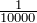23 = 8                 is equivalent to log2(8) = 3

52 = 25               is equivalent to log5(25) = 2

10-4 =is equivalent to log10() = -4

Example 4.2.2

Write these logarithmic equations as exponential equations: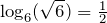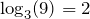is equivalent to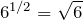is equivalent to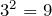By establishing the relationship between exponential and logarithmic functions, we can now solve basic logarithmic and exponential equations by rewriting.

Example 4.2.3

Solve log4(x) = 2 for x.

By rewriting this expression as an exponential, 42 = x , so x = 16

Most calculators and computers will only evaluate logarithms of two bases.

Common and Natural Logarithms:

The common log is the logarithm with base 10, and is typically written log(x) .

The natural log is the logarithm with base e, and is typically written ln(x) .

Example 4.2.4

Evaluate log(1000) using the definition of the common log.

To evaluate log(1000) , we can say x = log(1000) , then rewrite into exponential form using the common log base of 10.

10x = 1000

From this, we might recognize that 1000 is the cube of 10, so x = 3.

We also can use the inverse property of logs to write log10(103) = 3

 Values of the Common Log Number Number as exponential Log(number) 1000 103 3 100 102 2 10 101 1 1 100 0 0.1 10-1 -1 0.01 10-2 -2 0.001 10-3 -3

Example 4.2.5

Evaluate ln(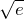) .
We can rewrite ln() as ln(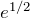) . Since ln is a log base e, we can use the inverse property for logs: ln() =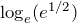=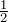.

# Graphs of Logarithms

Recall that the exponential function f(x) = 2x produces this table of values:

 x -3 -2 -1 0 1 2 3 f(x)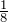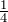1 2 4 8

Since the logarithmic function “undoes” the exponential, g(x) = log2(x) produces the table of values:

 x1 2 4 8 f(x) -3 -2 -1 0 1 2 3

In this second table, notice that:

1. As the input increases, the output increases.
2. As input increases, the output increases more slowly.
3. Since the exponential function only outputs positive values, the logarithm can only accept positive values as inputs, so the domain of the log function is (0, ∞) .
4. Since the exponential function can accept all real numbers as inputs, the logarithm can output any real number, so the range is all real numbers or (-∞, ∞) .

Sketching the graph, notice that as the input approaches zero from the right, the output of the function grows very large in the negative direction, indicating a vertical asymptote at x = 0. A vertical asymptote is a vertical line x = a where the graph tends towards positive or negative infinity as the inputs approach a.

In symbolic notation we write as x→0+ , f(x)→-∞ , and as x→∞ , f(x)→∞Graphical Features of the Logarithm

Graphically, in the function g(x) = logb(x):

• The graph has a horizontal intercept at (1, 0)
• The graph has a vertical asymptote at x = 0
• The graph is increasing and concave down
• The domain of the function is x > 0, or (0, ∞)
• The range of the function is all real numbers, or (-∞, ∞)

When sketching a general logarithm with base b, it can be helpful to remember that the graph will pass through the points (1, 0) and (b, 1). To get a feeling for how the base affects the shape of the graph, examine the graphs below.Notice that the larger the base, the slower the graph grows. For example, the common log graph, while it grows without bound, it does so very slowly. For example, to reach an output of 8, the input must be 100,000,000.

# Logarithm Properties

To utilize the common or natural logarithm functions to evaluate expressions like log2(10), we need to establish some additional properties.

Properties of Logs: Exponent Property

logb(Ar) = rlogb(A)

Example 4.2.6

Rewrite log3(25) using the exponent property for logs.

Since 25 = 52,

log3(25) = log3(52) = 2log35

Example 4.2.7

Rewrite 4ln(x) using the exponent property for logs.
Using the property in reverse, 4ln(x) = ln(x4)

The second important property allows us to change the base of a logarithmic expression.

Properties of Logs: Change of Base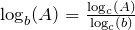Example 4.2.8

Evaluate log2(10) using the change of base formula.

According to the change of base formula, we can rewrite the log base 2 as a logarithm of any other base. Since our calculators can evaluate the natural log, we might choose to use the natural logarithm, which is the log base e: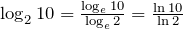Using our calculators to evaluate this: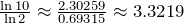This finally allows us to answer our original question – the population of flies we discussed at the beginning of the section will take 3.32 weeks to grow to 500.

Example 4.2.9

Evaluate log5(100) using the change of base formula.

We can rewrite this expression using any other base. We can rewrite using the common log, base 10: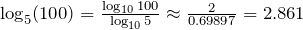An alternative approach to solving exponential equations is described below:

Solving exponential equations:

1. Isolate the exponential expressions when possible
2. Take the logarithm of both sides
3. Utilize the exponent property for logarithms to pull the variable out of the exponent
4. Use algebra to solve for the variable.

Example 4.2.10

Solve 2x = 10 for x.

Using this alternative approach, rather than rewrite this exponential into logarithmic form, we will take the logarithm of both sides of the equation. Since we often wish to evaluate the result to a decimal answer, we will usually utilize either the common log or natural log. For this example, we’ll use the natural log:

ln(2x) = ln(10)              Utilizing the exponent property for logs,

xln(2) = ln(10)              Now dividing by ln(2),

x =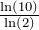≈ 2.861

Notice that this result matches the result we found using the change of base formula.

Example 4.2.11

Previously, we predicted the population (in billions) of India t years after 2008 by using the function f(t) = 1.14(1 + 0.0134)t . If the population continues following this trend, when will the population reach 2 billion?

We need to solve for the t so that f(t) = 2: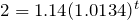Divide by 1.14 to isolate the exponential expression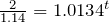Take the logarithm of both sides of the equation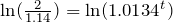Apply the exponent property on the right side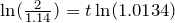Divide both sides by ln(1.0134)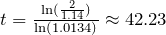years.

If this growth rate continues, the model predicts the population of India will reach 2 billion about 42 years after 2008, or approximately in the year 2050.

Some situations cannot be addressed using the properties already discussed. For these, we need some additional properties:

Sum of Logs Property: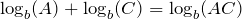Difference of Logs Property: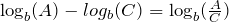With these properties, we can rewrite expressions involving multiple logs as a single log, or break an expression involving a single log into expressions involving multiple logs.

Example 4.2.12

Write log3(5) + log3(8) – log3(2) as a single logarithm.

Using the sum of logs property on the first two terms:

log3(5) + log3(8) = log3(5 · 8) = log3(40)

This reduces our original expression to log3(40) – log3(2)

Then using the difference of logs property,

log3(40) – log3(2) = log3(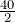) = log3(20)

Example 4.2.13

Evaluate 2log(5) + log(4) without a calculator by first rewriting as a single logarithm.

On the first term, we can use the exponent property of logs to write:

2log(5) = log(52) = log(25)

With the expression reduced to a sum of two logs, log(25) + log(4) , we can utilize the sum of logs property:

log(25) + log(4) = log(4 · 25) = log(100)

Since 100 = 102, we can evaluate this log without a calculator:

log(100) = log(102) = 2

Example 4.2.14

Rewrite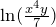as a sum or difference of logs.

First, noticing we have a quotient of two expressions, we can utilize the difference property of logs to write: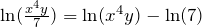Then seeing the product in the first term, we use the sum property: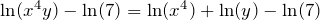Finally, we could use the exponent property on the first term: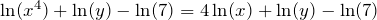# Log Properties in Solving Equations

The logarithm properties often arise when solving problems involving logarithms.

Example 4.2.15

Solve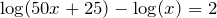.

In order to rewrite in exponential form, we need a single logarithmic expression on the left side of the equation. Using the difference property of logs, we can rewrite the left side: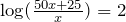Rewriting in exponential form reduces this to an algebraic equation: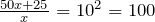Solving: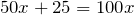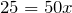Checking this answer in the original equation, we can verify there are no domain issues, and this answer is correct.

# Practice questions

1. Write the exponential equation 42 = 16 as a logarithmic equation.

2. Evaluate log(1,000,000).

3. Rewrite using the exponent property for logs: ln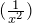.

4. Solve 5(0.93)x = 10.

5. Without a calculator evaluate by first rewriting as a single logarithm: log2(8) + log2(4)

6. Solve log(2x – 4) = 1 + log(x + 2).

7. Suppose the population of rats in a city is estimated to be 1.1 million. If the population size increases exponentially at a rate of 15% per year, how many years would it take for the population size to reach 5 million rats?